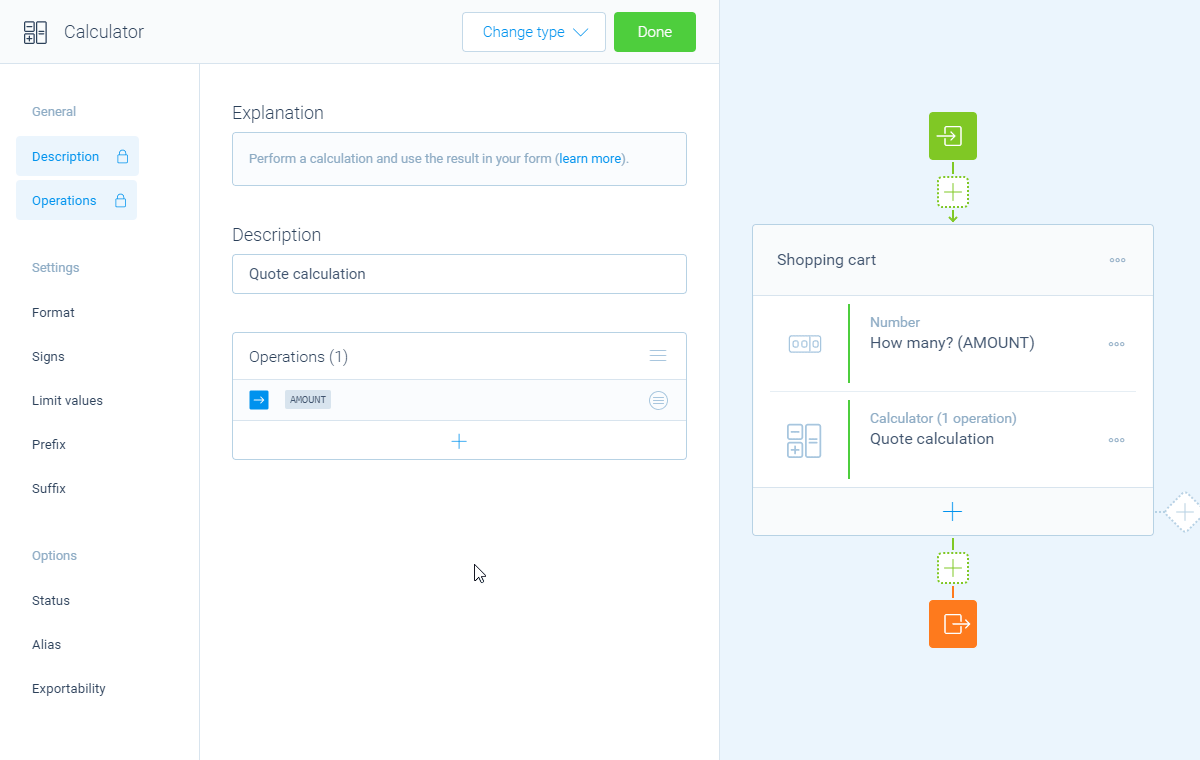Using logic4 Min.2 Min.

# How to use operations in the calculator block (add, subtract, multiply, divide, equal)

The calculator block supports all needed operations, like adding, subtracting, multiplying, dividing and equaling.

## When to use

Use the calculator block when you want to perform calculations inside your form. You will always need one or more operations to perform the needed calculations. For example:

• Add up the scores of correct given answers in a quiz;
• Subtract a discount in a quote form;
• Multiply a price of a product with the entered amount;
• Divide a value in a formula.

These are just some examples. Basically you can calculate anything you want with the calculator block. Please have a look at our calculator features overview to see everything you can do with the calculator block.

## How to use

Each calculation block starts with an empty list of `Operations`. These operations are the core of the calculator and determine what this block will calculate. Each operation is a step in your calculation. You can add as many operations as you need.

### Initial value

To start with, you add the `Initial value` to the list of operations by clicking the button at the bottom of the operations list. That's the starting point of your calculation.

There are several options to determine the initial value, for example static numbers, blocks, scores, comparisons, functions/constants and subcalculations.

### Operations

After you added the starting variable, you can perform all kinds of calculation operations to that. You can add unlimited operations by clicking the button at the bottom of the `Operations` list. The following operations are available:

• ` Add` - Add up a value to the outcome of the previous operation;
• ` Subtract` - Subtract a value from the outcome of the previous operation;
• ` Multiply` - Multiply the outcome of the previous operation with a value;
• ` Divide` - Divide the outcome of the previous operation with a value;
• ` Equal` - Equal the calculator with a value.

#### Operation properties

The properties of operations are so flexible, you can set them up exactly how you need them. It depends on what you want to do in the operation, what settings are available for that particular operation. Some operations don't need any additional settings, but others might have far-reaching options.

There are several options to use in the properties of your operation, for example static numbers, blocks (given answers), functions/constants and subcalculations.In this example we multiply the entered amount with the price (\$4.95/piece) and add the shipping costs (\$5.99).

#### Flexible values

The mindblowing thing with calculator operations is everything is totally flexible. Even if an operation needs some steps to get to the right value, all options are available in each step. For example a comparison has to take some steps to get to the right outcome. And in each step all possible options are usable:

• `Last outcome (ANS)`;
• `Number`Enter a static number;
• `Value (given answer)`Select a question from your form to use the answered value;
• `Constant`Select a built-in constant value.

### Management

You can use the following actions to manage your operations:

• You can click each operation to edit the properties. By clicking the button at the right side of each operation, you can alter the operator and delete a single operation;
• You can arrange the order of your operations by dragging and dropping a specific operation row. The operations get executed in the order that you set them in the operations list. That order of course can have a big effect on the outcome of the calculation;
• By clicking the button at the top of the operations list, you can reset the calculator by deleting the operations list.

The calculator block has lots of features, so we have several ways to learn all about it.

### Help center

Our help articles help you out on all different aspects of the calculator:

### Overviews

We also made some overviews of the capabilities that the calculator block provides: